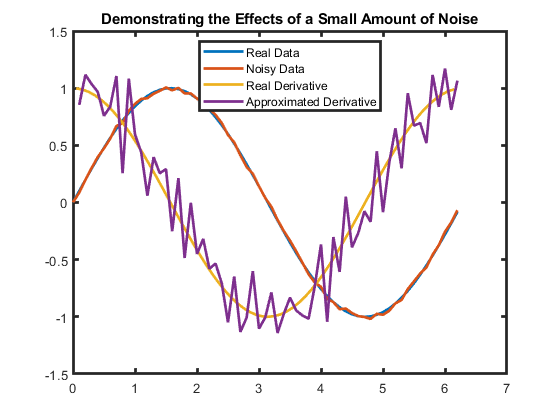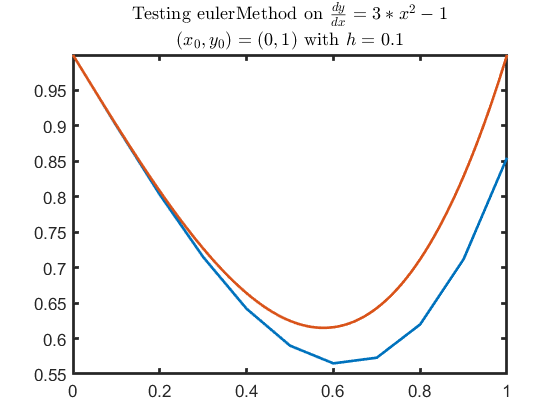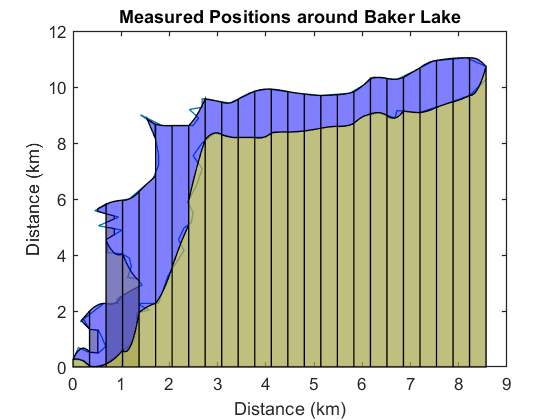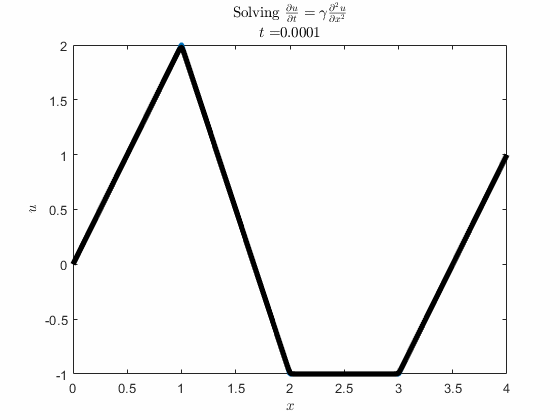## Numerical-Methods-with-Applications

version 1.0.3 (63.8 MB) by
Teach numerical methods for interpolation, differentiation, integration, and solving ODEs and PDEs with MATLAB.

Updated 3 Jun 2022

From GitHub

# Numerical Methods with ApplicationsCurriculum Module
Created with R2021b. Compatible with R2021b and later releases.

## Description

This curriculum module contains interactive live scripts that teach fundamental concepts and basic terminology related to designing and implementing numerical methods related to interpolation, numerical integration and differentiation, and numerical solutions to ordinary and partial differential equations.

These live scripts can be used as part of a lecture, as activities in an instructional setting, or as interactive assignments to be completed outside of class.

Get started with the Numerical Methods with Applications interactive examples by downloading and unzipping the repository. Then, double-click the NumericalMethods.prj file inside MATLAB®. From there, you can follow the landing page instructions to get started with the examples. The instructions inside the live scripts will guide you through the exercises and activities. Get started with each live script by running it one section at a time. To stop running the script or a section midway (for example, if a loop is running longer than intended), click the Stop button in the RUN section of the Live Editor tab in the MATLAB Toolstrip.

## Prerequisite Domain Knowledge

This module assumes familiarity with basic programming concepts such as floating-point doubles and strings, structures including constants, vectors, matrices, arrays, control flows including if/else, for loops, and while loops, and how to use them in MATLAB. These ideas are all presented with interactive examples in Fundamentals of Programming. This module assumes familiarity with polynomials, derivatives, and integrals. To use the partial differential equation scripts, a student must be familiar with the concepts of partial derivatives and multivariable functions. These scripts do not assume knowledge of analytic solutions to ordinary or partial differential equations.

## Details

NavigationOverview.mlx This script brings together links and short descriptions of the content in the recommended order of use.

Interpolation This folder includes the interactive example script `interpolation.mlx` that guides students through building their own data set(s) using `hand.mlx` and writing their own linear interpolation function and piecewise cubic Hermite interpolation function. The laboratory script `trackStorms.mlx` allows students to apply the scripts they have created to interpolate the paths of tropical cyclones from NOAA data.

Learning Goals:

• Define interpolation and explain how it is different from regression and extrapolation.
• Demonstrate multiple solutions to an interpolation problem, even when interpolating by polynomials.

Derivatives This folder includes the interactive example script `approximatingDerivatives.mlx` that introduces the method of Taylor series approximation to create and analyze numerical approximations to derivatives of different orders.Learning Goals:

• Determine numerical derivative approximations formulas.
• Use Taylor's theorem to calculate the order of the error for a numerical approximation to a derivative.
• Demonstrate how numerical derivatives can magnify approximation errors.

Integrals This folder includes the interactive example script `numericalIntegration.mlx` that guides students through building functions to compute forward and backward Euler method approximations, a 2-point Gaussian approximation, and a Simpson's rule approximation. The laboratory script `measureLake.mlx` presents two maps of a lake from different points in time and guides students through collecting data and realizing the complexities of numerically integrating from data.Learning Goals:

• Implement Euler's method, Gaussian 2-point approximations, and Simpson's rule for numerical integration.
• Explain why higher-order approximations may not be appropriate in applications.

Ordinary Differential Equations This folder includes the interactive example script `diffEqs.mlx` that guides students through implementing an Euler method solver and a four-step Runge-Kutta method solver, and comparing the results of their implementations to the built-in solver `ode45`. The laboratory script `pendulum.mlx` offers students a chance to apply numerical solution methods to create increasingly more realistic models of a simple pendulum.

Learning Goals:

• Implement Euler's method for first-order initial value problems.
• Implement a four-step Runge-Kutta method.

Partial Differential Equations This folder includes the interactive example script `partialDiffEqs.mlx` that guides students through an exploration of explicit, implicit, and mixed methods for numerically solving first-order ordinary differential equations. The activity script `implementExplicitSolver.mlx` provides scaffolded checks of each component required for creating `explicitPDE.m` to solve a first-order 1-D heat equation. The rest of `partialDiffEqs.mlx` builds upon `explictPDE.m` to create a related implicit method solver, a Crank-Nicolson solver, and a combined method solver.Learning Goals:

• Identify errors from discretizing the problem and from discretizing the method and choose appropriate parameters to minimize overall error.
• Explain the importance of stability when choosing a numerical method.
• Implement explicit, implicit, and Crank-Nicolson methods to solve a 1-D heat equation.

## Products

MATLAB®, Symbolic Math Toolbox™, Statistics and Machine Learning Toolbox™, Curve Fitting Toolbox™, Automated Driving Toolbox™

The license for this module is available in the LICENSE.TXT file in this GitHub repository.

## Support

The solutions are available upon instructor request. If you would like to request solutions or have a question, contact the MathWorks online teaching team.

### Cite As

Emma Smith Zbarsky (2022). Numerical-Methods-with-Applications (https://github.com/MathWorks-Teaching-Resources/Numerical-Methods-with-Applications/releases/tag/v1.0.3), GitHub. Retrieved .

##### MATLAB Release Compatibility
Created with R2021b
Compatible with R2021b and later releases
##### Platform Compatibility
Windows macOS Linux

### Community Treasure Hunt

Find the treasures in MATLAB Central and discover how the community can help you!

Start Hunting!

#### NumericalPDEs

To view or report issues in this GitHub add-on, visit the GitHub Repository.
To view or report issues in this GitHub add-on, visit the GitHub Repository.